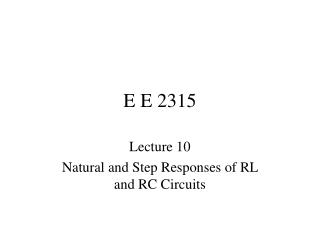DownloadDownload PresentationE E 2315

# E E 2315

Télécharger la présentation## E E 2315

- - - - - - - - - - - - - - - - - - - - - - - - - - - E N D - - - - - - - - - - - - - - - - - - - - - - - - - - -
##### Presentation Transcript

1. E E 2315 Lecture 10 Natural and Step Responses of RL and RC Circuits

2. Conservation of Charge (1/4) • Energy transferred if v10 v20 • Total system charge is conserved

3. Conservation of Charge (2/4) Initial stored energy: At equilibrium:

4. Conservation of Charge (3/4) Initial Charge: Final Charge: Since

5. Conservation of Charge (4/4) Final stored energy: Energy consumed in R:

6. Conservation of Flux Linkage (1/3) • Energy transferred if i10 i20 • Total system flux linkage is conserved. Initial stored energy: At equilibrium:

8. Conservation of Flux Linkage (3/3) Final stored energy: Energy consumed in R:

9. Natural RL Response (1/2) • Inductor has initial current, io. • Switch opens at t = 0 • Inductor current can’t change instantaneously

10. Natural RL Response (2/2) Integrate: KVL: Exponential of both sides: Separate the variables:

11. Natural RC Response (1/2) • Capacitor has initial voltage, vo. • Switch closes at t = 0. • Capacitor voltage can’t change instantaneously KCL: Separate the variables:

12. Natural RC Response (2/2) Integrate: Exponential of both sides:

13. RL Step Response (1/4) • Make-before-break switch changes from position a to b at t = 0. • For t < 0, Io circulates unchanged through inductor.

14. RL Step Response (2/4) • For t > 0, circuit is as below. • Initial value of inductor current, i, is Io. • The KVL equation provides the differential equation.

15. RL Step Response (3/4) Solution has two parts: Steady State Response Transient Response Determine k by initial conditions:

16. RL Step Response (4/4) • Inductor behaves as a short circuit to DC in steady state mode

17. RC Step Response (1/3) • Switch closes at t = 0. • Capacitor has initial voltage, Vo. v-i relationship: By KVL & Ohm’s Law:

18. RC Step Response (2/3) • Response has two parts • steady state • transient • Use initial voltage to determine transient Steady State Response Transient Response

19. RC Step Response (3/3) • Capacitor becomes an open circuit to DC after the transient response has decayed.

20. Unbounded Response (1/5) • Need Thévenin equivalent circuit from terminal pair connected to inductor • Let initial current = 0A in this example.

21. Unbounded Response (2/5) Voltage divider to get vx: Then Thévenin voltage

22. Unbounded Response (3/5) Therefore:

23. Unbounded Response (4/5) Steady state: Transient:

24. Unbounded Response (5/5) Use initial conditions to determine k. Complete response is unbounded: